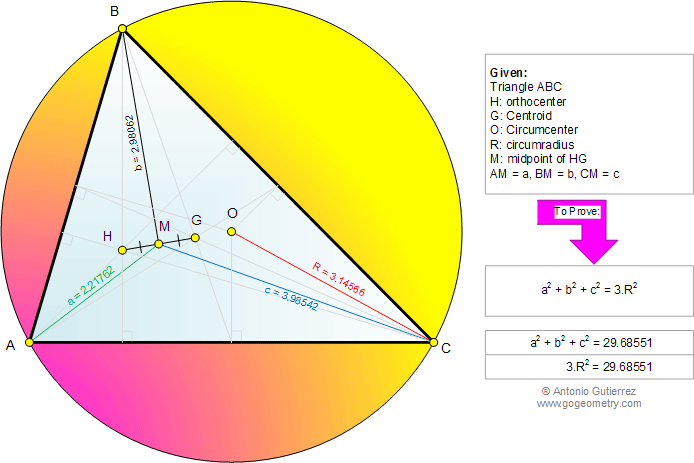# Online Geometry Problem 742: Scalene Triangle, Orthocenter, Centroid, Circumcenter, Circumradius, Midpoint, Distance, Square, Metric Relations. Level: High School, Honors Geometry, College, Mathematics Education

 The figure shows a triangle ABC with the orthocenter H and the centroid G. M is the midpoint of HG, AM = a, BM = b, CM = c, and R is the circumradius, Prove that a2 + b2 + c2 = 3.R2.Home | Search | Geometry | Problems | All Problems | Open Problems | Visual Index | Art | 741-750 | Triangles | Orthocenter | Centroid /Barycenter | Circumradius | Midpoints | Distances between Triangle Centers | Email | Solution / comment | by Antonio Gutierrez